# Two workers

One worker needs 40 hours to do a job, and the second would do it in 30 hours. They worked together for several hours, then the second was recalled, and the first completed the job itself in 5 hours. How many hours did they work together, and how much did each of them do?

Result

x =  15 h
a =  0.5
b =  0.5

#### Solution:

$\ \\ x \cdot \ (1/40+1/30) + 5/40 = 1 \ \\ \ \\ 7x = 105 \ \\ \ \\ x = 15 \ \\ = 15 \ \text { h }$
$a = \dfrac{ x+5 }{ 40 } = \dfrac{ 15+5 }{ 40 } = \dfrac{ 1 }{ 2 } = 0.5$
$b = \dfrac{ x }{ 30 } = \dfrac{ 15 }{ 30 } = \dfrac{ 1 }{ 2 } = 0.5$

Leave us a comment of this math problem and its solution (i.e. if it is still somewhat unclear...):Be the first to comment!#### Following knowledge from mathematics are needed to solve this word math problem:

Need help calculate sum, simplify or multiply fractions? Try our fraction calculator. Do you have a linear equation or system of equations and looking for its solution? Or do you have quadratic equation?

## Next similar math problems:

1. The touristThe tourist traveled 78km in 3 hours. Part of the journey went on foot at 6km/h, the rest of the trip by bus at 30km/h. How long did he walk?
2. Truck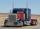Truck went for 4 hours and 30 minutes. If truck increase speed by 11 km/h, went same distance in 2 hours. Calculate this distance.
3. Two runners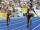Two runners ran simultaneously towards each other from locations distant 34.6 km. The average speed of the first runner was 1/5 higher than the average speed of the second runner. How long should each ran a 34.6 km, if you know that they meet after 67 mi
4. Fog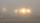The car started in fog at speed 30 km/h. After a 12-minute drive, the fog dissipated and the driver drove next 12 minutes distance 17 km. On the last 17 km long again the driving conditions deteriorated and the driver drove the speed of 51 km/h. a) Calcu
5. Tourists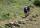From the cottage started first group of tourists at 10:00 AM at speed 4 km/h. The second started after them 47 minutes later at speed 6 km/h. How long and how many kilometers from the cottage will catch the first group?
6. Filament of bulbThe filament of bulb has a 1 ohm resistivity and is connected to a voltage 220 V. How much electric charge will pass through the fiber when the electric current passes for 10 seconds?
7. ResistanceDetermine the resistance of the bulb with current 200 mA and is in regular lamp (230V).
8. Coil as a girl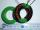The electrical resistance of the copper wire coil is 2.0 ohms. What current runs through the coil when the voltage between the terminals is 3.0 V?
9. TransformerSolve the textbook problems - transformer: a) N1 = 40, N2 = 80, U2 = 80 V, U1 =? b) N1 = 400, U1 = 200 V, U2 = 50 V, N2 =?
10. Resistance of the resistorThe resistor terminals have a voltage of 20 V and a current of 5 mA is passed through. What is the resistance of the resistor?
11. Voltmeter range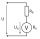We have a voltmeter which in the original set measures voltage to 10V. Calculate the size of the ballast resistor for this voltmeter, if we want to measure the voltage up to 50V. Voltmeter's internal resistance is 2 kiloohm/Volt.
12. Z9–I–1In all nine fields of given shape to be filled natural numbers so that: • each of the numbers 2, 4, 6 and 8 is used at least once, • four of the inner square boxes containing the products of the numbers of adjacent cells of the outer square, • in the cir
13. Reconstruction of the corridorCalculate how many minutes will be reduced to travel 187 km long railway corridor, where the maximum speed increases from 120 km/h to 160 km/h. Calculate how many minutes will shorten travel time, if we consider that the train must stop at 6 stations, eac
14. Square gridSquare grid consists of a square with sides of length 1 cm. Draw in it at least three different patterns such that each had a content of 6 cm2 and circumference 12 cm and that their sides is in square grid.
15. Hexagon - MOThe picture shows the ABCD square, the EFGD square and the HIJD rectangle. Points J and G lie on the side CD and is true |DJ|
16. Train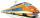The train is running at speeds of 96 km/h. From the beginning of braking to full stop train run for 3.3 minutes. If the train slows the braking equally, calculate the distance of the place from the station where you need to start to brake.
17. Average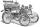What is the average speed of the car, where half of the distance covered passed at speed 66 km/h and the other half at 86 km/h.